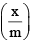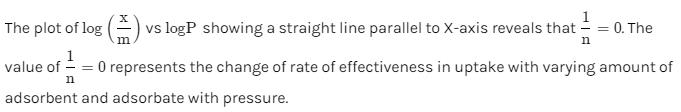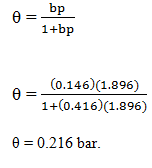Courses

# Test: Adsorption - 1

## 12 Questions MCQ Test Physical Chemistry | Test: Adsorption - 1

Description
This mock test of Test: Adsorption - 1 for Chemistry helps you for every Chemistry entrance exam. This contains 12 Multiple Choice Questions for Chemistry Test: Adsorption - 1 (mcq) to study with solutions a complete question bank. The solved questions answers in this Test: Adsorption - 1 quiz give you a good mix of easy questions and tough questions. Chemistry students definitely take this Test: Adsorption - 1 exercise for a better result in the exam. You can find other Test: Adsorption - 1 extra questions, long questions & short questions for Chemistry on EduRev as well by searching above.
QUESTION: 1

Solution:
QUESTION: 2

Solution:
QUESTION: 3

### Which statement is not correct:

Solution:
QUESTION: 4

Which is correct in case of van der Waals’ adsorption:

Solution:

If the accumulation of gas on the surface of a solid occurs on account of weak van der Waals’ forces, the adsorption is termed as physical adsorption or physisorption.

Hence, Physisorption can also be called as Van der Waals adsorption.

Physical adsorption of a gas by a solid is generally reversible. We can say by Le-Chatelier's principle that more of gas is adsorbed when pressure is increased as the volume of the gas decreases and since the adsorption process is exothermic, the physical adsorption occurs readily at low temperature and decreases with increasing temperature.

So the correct case is when it is Low temperature and high pressure the Physisorption tends to happen.

QUESTION: 5

According to Langmuir adsorption isotherm the amount of gas adsorbed at very high pressure:

Solution:
QUESTION: 6

Which characteristic is not correct for physical adsorption:

Solution:
QUESTION: 7

Rate of physisorption increases with:

Solution:
QUESTION: 8

Adsorption of a gas on solid metal surface is spontaneous and exothermic, then:

Solution:
QUESTION: 9

Surface area available for adsorption per g of catalyst is called:

Solution:
QUESTION: 10

Plots of logvs. log C showing a straight line parallel to X-axis reveals that:

Solution:QUESTION: 11

The Langmuir adsorption isotherm is deduced using the assumption:

Solution:
QUESTION: 12

Adsorption of methane follows the Langmuir adsorption isotherm at 90K. If p = 1.896cm3g-1bar-1 and b = 0.146bar-1. Calculate the value of θ.

Solution:

Given data
p = 1.896cm3g-1 bar-1
b = 0.146 bar-1

Substitute in the corresponding equation